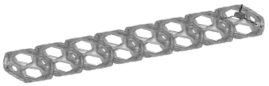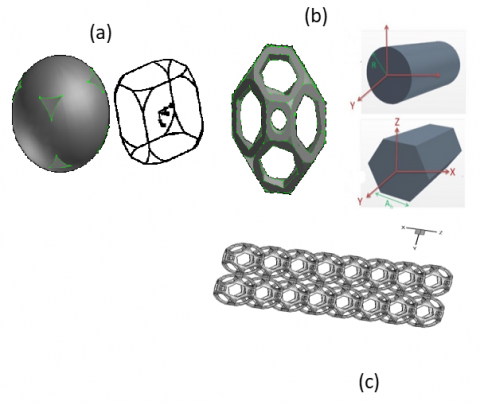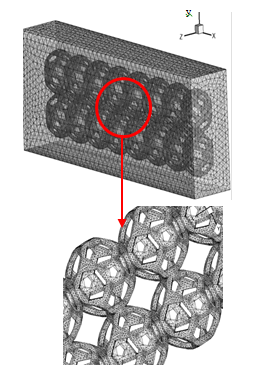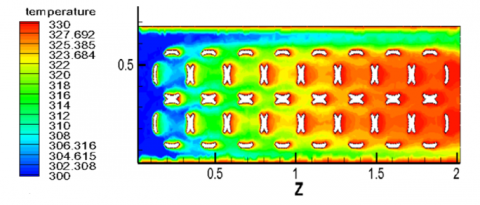# Numerical Simulation of Air Flow and Temperature Distribution in Volumetric Solar Receiver Consisting of a Porous Medium

Numerical Simulation of Air Flow and Temperature Distribution in Volumetric Solar Receiver Consisting of a Porous Medium
L.P.E.A. Laboratory-Matter Sciences Faculty, University of Batna 1, Batna 05000, Algeria

Department of Physics-Matter Sciences Faculty-L.P.E.A. Laboratory, University of Batna 1, Batna 05000, Algeria

Corresponding Author Email:
sihem.djouimaa@univ-batna.dz
Page:
459-464
|
DOI:
https://doi.org/10.18280/mmep.070317
14 April 2020
|
Accepted:
10 August 2020
|
Published:
30 September 2020
| Citation

OPEN ACCESS

Abstract:

This study presents a numerical analysis of the flow of fluids and the temperature distribution in a volumetric solar receiver which is considered as a porous medium. This medium consists of ceramic foams with regular and periodic structures. The study is based on the resolution of the mean and three-dimensional Reynolds unsteady equations. The SST K - w model is used to visualize the effects of turbulence. This study was carried out on the Tetrakaidecahedra structure with a high tortuous shape which causes very large speeds and temperature gradients. We were able to reach temperature equilibrium for different Reynolds numbers. The results of the pressure drops were compared to the predicted models using other different structures. The results give us very good agreement by comparing them with the data from the literature.

Keywords:

ceramic foams, local temperature equilibrium, porous medium, tetrakaidecahedra structure turbulence, volumetric solar receiver

1. Introduction

Ceramics are materials with very favorable thermophysical properties, they are mainly used for the design of volumetric receivers for their high resistance to strong temperature gradients. Their plastic deformation is low and their melting temperature is very high (up to 2000℃), their density is low with a strong endurance. For that, we are principally interested to study air flow and convective heat transfer in a solar receiver constituted of a ceramic porous medium. This medium is presented by a series of periodic (3D) structures, in which, one element is constructed according to the Kelvin model.

Certainly, the radiations generated from heliostats field are focalized to the solar receiver, in which the heat is transferred from the solid phase to the air as it passes through the porous medium that absorb solar radiation in depth. The domain of receivers presents a great interest for a green electricity production, in this way, many researchers invested their efforts to increase the efficiency of porous media in volumetric receivers. Most of the works are concentrated in the study of heat transfer phenomena and pressure drop parameters in open cell foams numerically and analytically for different open cell foams with randomized forms. The medium has tortuosity’s which allow the increase of the heat exchange, and the reduction of the pressure losses compared to packed bed of spheres Kumar et al. .

Different forms of ceramic foams geometries have been a subject of thermic and dynamic studies; we cite for example, the cubic model of Dul’nev Lacroix  and Krishnan , Weaire–Phelan’s unit cell , Kelvin’s tetrakaidecahedral model Boomsma , Richardson , and 3D Laguerre-Voronoi model studied by Nie et al. . The Kelvin’s model is widely used because of its useful construction.

Among the works done on the kelvin structure (Tetrakaidecahedra), Lucci et al.  presented a parametric CFD analysis of Kelvin cell structures to study foams in a controlled environment. In particular their results are about 20% higher than that predicted by the correlation of Groppi et al. . Zhang  proposed a modified Kelvin model for high mechanical property open-cell metal foams and investigated its application in thermal simulations. Ferrari et al.  analyzed three-dimensional fluid dynamics in order to evaluate the convective heat transfer coefficient in regular and non-regular structures. They also evaluated the pressure drop to extract the best performance given by the different structures.

Other research was focused on numerical simulations based on detailed foam structure models to predict effective properties of porous foams associated with fluid flow and/or heat transfer. Kuwahara et al.  studied numerically the interfacial convective heat transfer coefficient using a single structural unit. Petrasch et al.  determined the permeability, Dupuit-Forchheimer coefficient, and interfacial heat transfer coefficient in 10 ppi porous ceramic through a 3D digital sample representation generated from X-ray tomographic scans. They solved the governing equations of fluid flow via the finite volume direct pore-level numerical simulation. Mendes et al.  provided a simplified modeling approach for estimating the effective thermal conductivity of open cell foam using a finite volume method. Wu et al.  simulated numerically the convective heat transfer in ceramic foams represented by an idealized packed tetrakaidecahedra structure. Xu et al.  used the numerical method to analyze the typical influences of the porosity, average particle diameter and inlet velocity on the temperature distributions.

This work has as a principal objective, to participate in the performance of the kelvin structure in order to use it in the volumetric solar receiver. The main equations (continuity, momentum and energy) are solved simultaneously to determine dynamic and heat transfer characteristics between the ﬂowing ﬂuid and ceramic foam which is a periodic packed tetrakaidecahedron regular structure. The SSTkw turbulent model is used to appropriate the predict simulation. According to Menter et al. [17, 18], Vijay Garg and Ameri  this model gives results with high quality for a variety of heat transfer cases. Instationary and three-dimensional simulations are realised with the FLUENT software package. Calculations are made for one and two rows of structures. Our validation is based on the comparison with data and results provided in Wu et al. .

2. Physics Models Description

2.1 Geometry

The geometry studied in this work consists of a parallelepiped channel of length L = 1m, width and height equal to 60 mm in which is placed ceramic foams.  They can be described as a series of three dimensional (3D) periodic structures. One element is constructed according to the Kelvin model. (Figure 1). Two geometries cases are presented: the first one is a square duct with one row and eight structures (Figure 1-a). The second one is composed with two rows and sixteen structures (Figure 1-b); which dimensions are taken from Wu et al. .

## 1a.png(a)

## 1b.png(b)

Figure 1. Studied geometry

2.2 Geometry conception

The proposed model foam structures are created using the pre-processor GAMBIT. We first star with creating spheres with specified size distribution packed into a cubed volume (Figure 2-a), after substraction, the foam struts are created by using cylinders and prismes  with defined constant diameter (Figure 2-b) Once we have the main element obtained, we proceed to the construction of structures starting from this last one to form different geometries (Figure 2-c), it should be noted that this method of construction is very complicated and the meshing procedure was very challenging because of the complexity of the foam structures.

## 2.pngFigure 2. Model of constructed geometry

3. Governing Equations

In porous media, in order to use the Navier Stokes equations, it is necessary to have a saturated medium, the principal formulas used to resolve the problem are derived from the second law of Newton and the first principle of thermodynamics.

The conservation of mass equation for a control volume in differential form is defined as:

$\frac{\partial \rho}{\partial \mathrm{t}}+\frac{\partial\left(\rho \mathrm{U}_{\mathrm{j}}\right)}{\partial \mathrm{x}_{\mathrm{j}}}=0$     (1)

The conservation of momentum in a static reference frame is given by:

$\frac{\partial\left(\rho \mathrm{U}_{\mathrm{i}}\right)}{\partial \mathrm{t}}+\frac{\partial\left(\rho \mathrm{U}_{\mathrm{i}} \mathrm{U}_{\mathrm{j}}\right)}{\partial \mathrm{x}_{\mathrm{j}}}=-\frac{\partial \mathrm{P}}{\partial \mathrm{x}_{\mathrm{i}}}$$+\frac{\partial}{\partial x_{j}}\left(\mu\left(\frac{\partial U_{i}}{\partial x_{j}}+\frac{\partial U_{j}}{\partial x_{i}}\right)-\left(\frac{2}{3} \delta_{i j} \mu \frac{\partial U_{k}}{\partial x_{k}}\right)-\rho \overline{u_{i} u_{j}}\right)$   (2)

The energy equation is presented by:

$\frac{\partial(\rho \mathrm{E})}{\partial \mathrm{t}}+\frac{\partial\left(\rho \mathrm{U}_{\mathrm{j}} \mathrm{E}\right)}{\partial \mathrm{x}_{\mathrm{j}}}=\frac{\partial}{\partial \mathrm{x}_{\mathrm{j}}}\left(\lambda_{\mathrm{c}}\left(\frac{\partial \mathrm{T}}{\partial \mathrm{x}_{\mathrm{j}}}\right)+\mathrm{U}_{\mathrm{i}} \tau_{\mathrm{ij}}-\rho \mathrm{C}_{\mathrm{v}} \overline{\mathrm{u}_{i} \mathrm{T}^{\prime}}\right)$     (3)

$E=h-\frac{p}{\rho}+\frac{U_{i}^{2}}{2}, \frac{p}{\rho}=R T$     (4)

4. Numerical Method

The governing equations are solved with a finite volume scheme using the code Fluent. The second order is automatically adopted for almost variables (Pressure, Momentum, Turbulent kinetic energy equation and its dissipation Rate), The simple scheme is applied to Pressure-velocity coupling. The residuals (continuity equation, velocity, turbulent kinetic energy K and Omega) are at 10-4 and the energy is at 10-6.

4.1 Mesh presentation

To capture the resulted temperature-velocity gradients for accurately simulation of the turbulent flow and heat transfer a quality grid is needed, especially near the structures. The irregular structured grid is generated by the pre-processor GAMBIT, which is obtained using the tetra hybrid scheme. The final grid used in this paper was approximately 13520 elements (Figure 3).

## 3.pngFigure 3. Computational grid

## 4.pngFigure 4. The geometry with boundary conditions

4.2 Boundary conditions

Figure 4 show the boundary conditions imposed to the computational domain. At the inlet, a constant velocity was imposed with values ranging from 0.5 to 5 m/s, and static temperature equal to 300 K, a pressure-outlet boundary condition with zero-gauge pressure is used at the outlet. The strut surfaces were defined as non-slip walls with a constant temperature of 330 K and the lateral walls of the rectangular duct are taken as symmetry conditions Wu et al. .

5. Results and Discussion

To carry out this study, we took into account the variations of the following parameters: Reynolds number effect at the entrance and the porosity of a part of the computational domain that is presented by two rows of the matrix structure (Figure 4). The fluid enters the conduit, with an ambient temperature Tf equal to 300 K, by interacting with the structures of the source of the domain which are at the temperature TS equal to 330 K. During this interaction the fluid extracts a quantity of heat until reaching the local equilibrium temperature (the temperature of the fluid is equal to that of the solid Ts = Tf).

5.1 Grid dependence

Because of the foam shape and the complex geometry, the flow is very tortuous; which generates strong temperature and speed gradients, so here we have to present a high-quality mesh. A study of the mesh sensitivity was conducted by checking the temperature which is a very sensitive parameter.

## 5.pngFigure 5. Grid dependence: Dimensionless temperature profiles

Figure 5 shows the dimensionless profiles of the temperature (Ts-Tf) /(Ts-Tfin)  for two grids; (13520) and (346485). The superposition of these profiles shows a very small difference (3.8%) between the values. Therefore, we opted for the first mesh to reduce the computation time.

5.2 Temperature behavior

The first results present the temperature distribution along the direction of flow. The air begins to extract the thermal energy from the structures until it reaches the local equilibrium temperature, which has been represented by the middle plane of the thermal field (Figure 6) and (Figure 7).

## 6.pngFigure 6. The temperature field along the flow direction

## 7.pngFigure 7. Variation of static temperature with horizontal position Z, Re =129

## 8.pngFigure 8. Temperature profiles with different velocities

## 9.pngFigure 9. Temperature profiles with one and two rows

Figure 8 thus presents the behavior in term of the temperature of the air in the matrix with varying inlet velocities. The elevated flow rates allow better temperature diffusion, with a distinct strong energy extraction. When the contact between the air and the structures lasts longer, the energy transfer is better and the fluid reaches the equilibrium temperature at a distance very far from the outlet.

We note that low velocities allow the extraction of energy more than the high ones. The velocity inlet (Reynolds number) affects directly the temperature behavior in the matrix medium .

In order to optimize the solar receiver geometry as mentioned before, we expose the results of the second geometry with two rows and compare them with the one’s one Figure (1-a, 1-b). We note that the fluid reaches the temperature of the solid in a very advanced position for the case of two rows, unlike for one row (The half of the first distance); where the propagation of heat generates a thermal equilibrium in position far downstream (Figure 9).

5.3 Pressure and velocity behavior

The shape of the structure of the Kelvin matrix causes irregularities which appear in the latter. The particles of fluid meet regions of separation and re-attachment which are at the origin of the dissipation of the kinetic energy which will be amplified by strong gradients of speed and temperature. (Figure 10) shows streamlines colored by velocity in the symmetric plane along the z axis.

## 10.pngFigure 10. Streamlines colored by velocity

This shows that there is a separation in streamlines in the foam, which is observed in the work of Nie et al. . The majority of current lines encounter an impact zone in the foam, and depending on the speed - temperature gradients producing, the phenomena generated, favor the transfer by convection and rapid diffusion of heat into the fluid medium.

The Figure (11.a) shows the distribution of the mean velocity along the flow direction. Although the Reynolds number is small, the flow always remains turbulent because of the continuous change of the directions of the particles and the penetration in the pores by causing accelerations according to the conservation of the mass, this phenomenon is observed by  Nie et al. . The same remarks are also observed in other studies, for example [20, 21]. A larger cross section of the spacer can cause a greater separation effect, and therefore a greater reduction in heat transfer.

The effects of friction indicate an internal heat generation followed by a reduction of the density of the fluid, to make pass the same mass flow, the continuity forces the velocity to increase and automatically the static pressure to decrease (Figure 11.b).

## 11.pngFigure 11. Velocity (a) and Pressure field (b)

According to Nie et al. , the complex structure of pores causes a complex pressure distribution inside the foam and stagnation areas are observed in front of the struts.

## 12.pngFigure 12. Static pressure profiles: one and two rows

Figure 12 presents the static pressure profile with one and two rows, which decreases linearly. The presence of more structures causes a high pressure drop. However, the decreasing porosity generates an increasing pressure drop.

The Figure 13 presents a comparison between Ergun correlation calculating the pressure drop and our simulation results concerning the same parameter.

The pressure drop increases with increasing velocity.  Compared with Ergun’s model, the predicted value agrees well at a velocity of 3.4 m/s. The curve follows the same draft as the proposed model, larger in the range of 1<V< 3.4 and smaller for V > 3.4 It can be noted that the simulated data are in good agreement With an Ergun's  model because it is the most successful model for predicting the pressure drop of porous medium  especially for high porosities: The Kelvin structure porosity is equal to 0.92.

• model 1 is a correlation of Ergun. :

$\frac{\mathrm{dp}}{\mathrm{dz}}=\frac{(1-\varepsilon)^{2} \mu \mathrm{V}}{150 \mathrm{d}^{2} \varepsilon^{3}}+\frac{(1-\varepsilon) \rho \mathrm{V}^{2}}{1.75 \mathrm{d} \varepsilon^{3}}$      (5)

## 13.pngFigure 13. Pressure drop comparison for one row and velocity varieties, our simulations and theoretical prediction Ergun 

6. Conclusion

In this study, a turbulent air flow in ceramic foams proposed to use it in volumetric receiver was performed. The effects of the geometric parameters, on the air flow in the porous medium, were investigated. The pressure drop of open cell foams was studied on foam structures. The conclusions drawn in this work are summarized as follows:

The pressure of open cell foams linearly decreases along the flow direction and the velocity field presents large velocity fluctuations throughout the entire porous medium. The mean fluid temperature increases along the flow direction. The equilibrium temperature is early reached for small velocities. The two rows allow us to optimize the receiver length (50%). The difference between laminar and turbulent flow is negligible.

Nomenclature
 CP specific heat at constant pressure, J. kg-1. K-1 d Mean pore diameter, m dp Particle diameter, m h Enthalpy, J/Kg K Thermal conductivity, W/m K P Pressure, Pa Sv Specific surface area, m2/m3 T temperature, K t Time, s U,V Velocity, m/s vi, vj Velocity in the I and j direction respectively, m/s xi, xj Coordinates in the I and j direction respectively, m/s Greek symbols $\Delta \mathrm{P}$ Pressure drop, Pa ɛ Porosity $\mu$ Dynamic viscosity, kg. m-1.s-1 u Kinematic viscosity, m2/s ρ Density, kg/m3 Subscripts f Fluid (air) s Solid (ceramic)
References

 Kumar, P., Biswas, S., Kumari, S. (2014). Building integrated photovoltaic generation system. In 2014 1st International Conference on Non Conventional Energy (ICONCE 2014), pp. 80-83. https://doi.org/10.1109/ICONCE.2014.6808688

 Lacroix, M., Nguyen, P., Schweich, D., Huu, C.P., Savin-Poncet, S., Edouard, D. (2007). Pressure drop measurements and modeling on SiC foams. Chemical Engineering Science, 62(12): 3259-3267. https://doi.org/10.1016/j.ces.2007.03.027

 Krishnan, S., Murthy, J.Y., Garimella, S.V. (2006). Direct simulation of transport in open-cell metal foam. Journal of Heat Transfer, 128(8): 793-799. http://dx.doi.org/10.1115/1.2227038

 Boomsma, K., Poulikakos, D., Ventikos, Y. (2003). Simulations of flow through open cell metal foams using an idealized periodic cell structure. International Journal of Heat and Fluid Flow, 24(6): 825-834. https://doi.org/10.1016/j.ijheatfluidflow.2003.08.002

 Boomsma, K., Poulikakos, D. (2001). On the effective thermal conductivity of a three-dimensionally structured fluid-saturated metal foam. International Journal of Heat and Mass Transfer, 44(4): 827-836. https://doi.org/10.1016/S0017-9310 (00)00123-X

 Richardson, J.T., Peng, Y., Remue, D. (2000). Properties of ceramic foam catalyst supports: Pressure drop. Applied Catalysis A: General, 204(1): 19-32. https://doi.org/10.1016/S0926-860X(00 )00508-10

 Nie, Z., Lin, Y., Tong, Q. (2017). Numerical investigation of pressure drop and heat transfer through open cell foams with 3D Laguerre-Voronoi model. International Journal of Heat and Mass Transfer, 113: 819-839. https://doi.org/10.1016/j.ijheatmasstransfer.2017.05.119

 Lucci, F., Della Torre, A., von Rickenbach, J., Montenegro, G., Poulikakos, D., Eggenschwiler, P.D. (2014). Performance of randomized Kelvin cell structures as catalytic substrates: Mass-transfer based analysis. Chemical Engineering Science, 112: 143-151. https://doi.org/10.1016/j. ces. 2014.03.023

 Groppi, G., Giani, L., Tronconi, E. (2007). Generalized correlation for gas/solid mass-transfer coefficients in metallic and ceramic foams. Industrial & Engineering Chemistry Research, 46(12): 3955-3958. https://doi.org/10.1021/ie061330g

 Zhang, C. (2015). A modified Kelvin model for thermal performance simulation of high mechanical property open-cell metal foams. Journal of Materials Science and Chemical Engineering, 3(7): 113-118. http://dx.doi.org/10.4236/msce.2015.37015

 Ferrari, L., Barbato, M.C., Ortona, A., D’Angelo, C. (2014). Convective heat transfer in cellular ceramic: A 3D numerical solution. International Conference on Heat Transfer, Fluid Mechanics and Thermodynamics. Orlando, Florida.

 Kuwahara, F., Shirota, M., Nakayama, A. (2001). A numerical study of interfacial convective heat transfer coefficient in two-energy equation model for convection in porous media. International Journal of Heat and Mass Transfer, 44(6): 1153-1159. https://doi.org/10.1016/S0017310(00) 00166-6

 Petrasch, J., Meier, F., Friess, H., Steinfeld, A. (2008). Tomography based determination of permeability, Dupuit–Forchheimer coefficient, and interfacial heat transfer coefficient in reticulate porous ceramics. International Journal of Heat and Fluid Flow, 29(1): 315-326. https://doi.org/10.1016/j.ijheatfluidflow.2007.09.001

 Mendes, M.A., Ray, S., Trimis, D. (2013). A simple and efficient method for the evaluation of effective thermal conductivity of open-cell foam-like structures. International Journal of Heat and Mass Transfer, 66: 412-422. https://doi.org/10.1016/j.ijheatmasstransfer.2013.07.032

 Wu, Z., Caliot, C., Flamant, G., Wang, Z. (2011). Numerical simulation of convective heat transfer between air flow and ceramic foams to optimise volumetric solar air receiver performances. International Journal of Heat and Mass Transfer, 54(7-8): 1527-1537. https://doi.org/10.1016/j.ijheatmasstransfer.2010.11.037

 Xu, C., Song, Z., Zhen, Y. (2011). Numerical investigation on porous media heat transfer in a solar tower receiver. Renewable Energy, 36(3): 1138-1144. https://doi.org/ 10.1016/j.renene.2010.09.017

 Menter, F.R., Kuntz, M., Langtry, R. (2003). Ten years of industrial experience with the SST turbulence model. Turbulence, Heat and Mass Transfer, 4(1): 625-632.

 Menter, F., Vieser, W., Esch, T. (2002). Heat transfer predictions using advanced two-equation turbulence models. CFX Validation Report.

 Garg, V.K., Ameri, A.A. (2001). Two-equation turbulence models for prediction of heat transfer on a transonic turbine blade. American Society of Mechanical Engineers, 78521, V003T01A044.

 Kopanidis, A., Theodorakakos, A., Gavaises, E., Bouris, D. (2010). 3D numerical simulation of flow and conjugate heat transfer through a pore scale model of high porosity open cell metal foam. International Journal of Heat and Mass Transfer, 53(11-12): 2539-2550. https://doi.org/10.1016/j.ijheatmasstransfer.2009.12.067

 Iasiello, M., Cunsolo, S., Bianco, N., Chiu, W.K., Naso, V. (2017). Developing thermal flow in open-cell foams. International Journal of Thermal Sciences, 111: 129-137. https://doi.org/10.1016/j.ijthermalsci.2016.08.013

 Ergun, S. (1952). Fluids flow through packed columns. Carnegie burgh, Pennsylvania.

 Mancin, S., Zilio, C., Diani, A., Rossetto, L. (2012). Experimental air heat transfer and pressure drop through copper foams. Experimental Thermal and Fluid Science, 36: 224-232. https://doi.org/10.1016/j.expthermflusci.2011.09.016